# Datalog S Costantini stefcostdi univaq it Engineering Ig

• Slides: 55Datalog S. Costantini [email protected] univaq. it Engineering Ig. Technology Maggioli Informatica Micron Technology Neta Nous Informatica Nikesoft SED Siemens CNX Taiprora Telespazio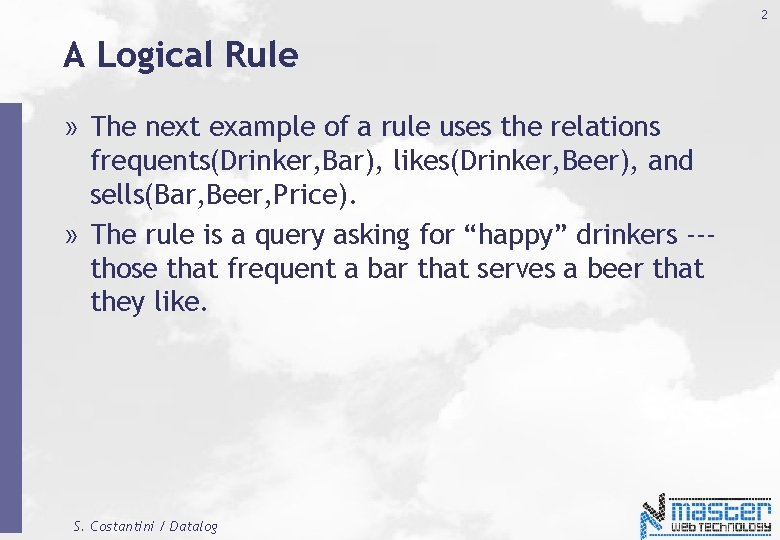2 A Logical Rule » The next example of a rule uses the relations frequents(Drinker, Bar), likes(Drinker, Beer), and sells(Bar, Beer, Price). » The rule is a query asking for “happy” drinkers --those that frequent a bar that serves a beer that they like. S. Costantini / Datalog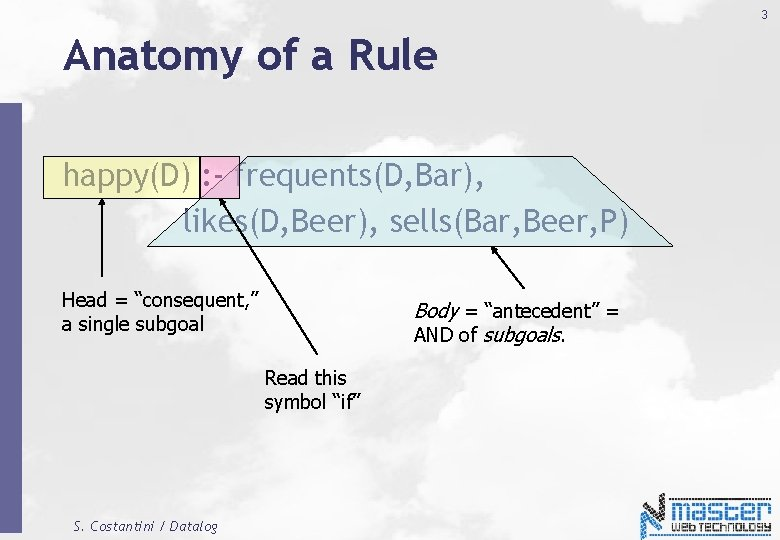3 Anatomy of a Rule happy(D) : - frequents(D, Bar), likes(D, Beer), sells(Bar, Beer, P) Head = “consequent, ” a single subgoal Body = “antecedent” = AND of subgoals. Read this symbol “if” S. Costantini / Datalog4 Subgoals Are Atoms » An atom is a predicate, or relation name with variables or constants as arguments. » The head is an atom; the body is the AND of one or more atoms (AND denoted by “, ”). S. Costantini / Datalog5 Example: Atom sells(Bar, Beer, P) The predicate = name of a relation S. Costantini / Datalog Arguments are variables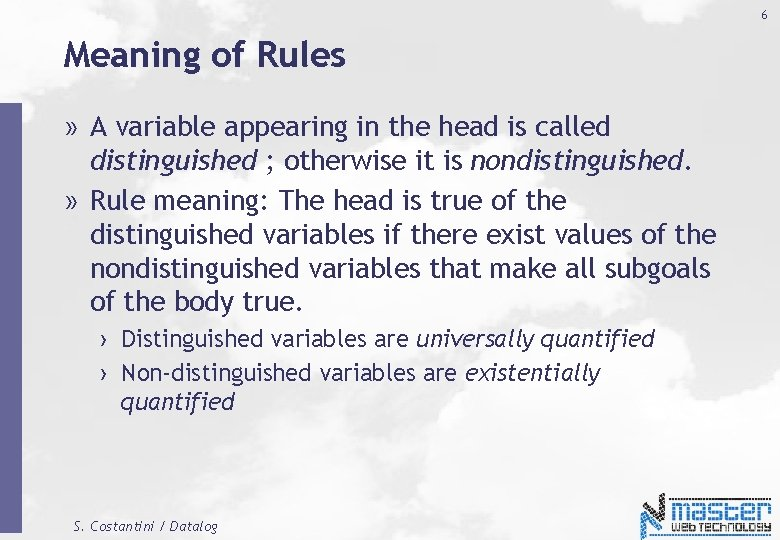6 Meaning of Rules » A variable appearing in the head is called distinguished ; otherwise it is nondistinguished. » Rule meaning: The head is true of the distinguished variables if there exist values of the nondistinguished variables that make all subgoals of the body true. › Distinguished variables are universally quantified › Non-distinguished variables are existentially quantified S. Costantini / Datalog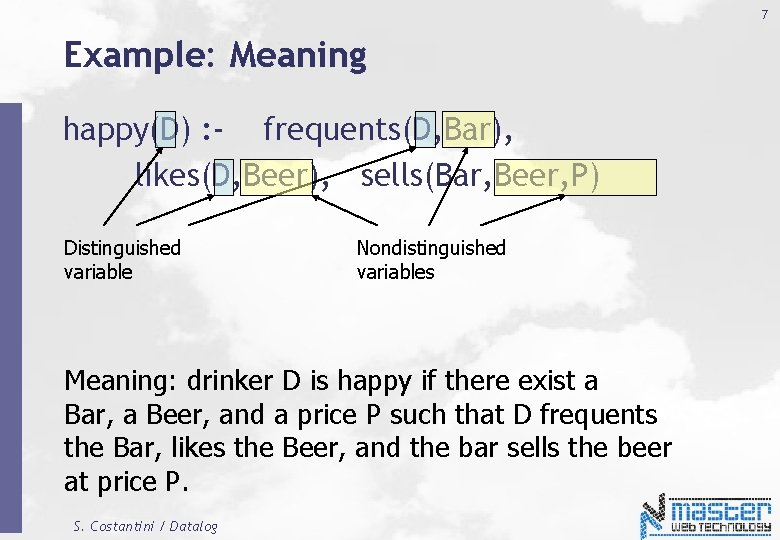7 Example: Meaning happy(D) : - frequents(D, Bar), likes(D, Beer), sells(Bar, Beer, P) Distinguished variable Nondistinguished variables Meaning: drinker D is happy if there exist a Bar, a Beer, and a price P such that D frequents the Bar, likes the Beer, and the bar sells the beer at price P. S. Costantini / Datalog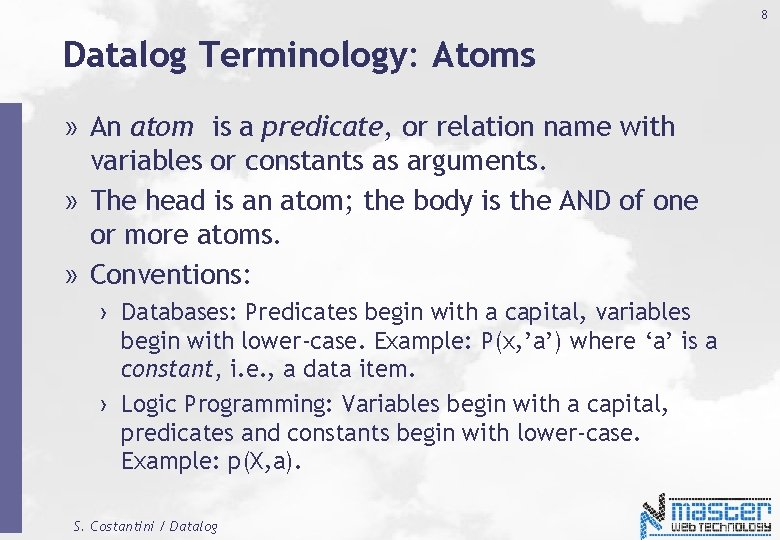8 Datalog Terminology: Atoms » An atom is a predicate, or relation name with variables or constants as arguments. » The head is an atom; the body is the AND of one or more atoms. » Conventions: › Databases: Predicates begin with a capital, variables begin with lower-case. Example: P(x, ’a’) where ‘a’ is a constant, i. e. , a data item. › Logic Programming: Variables begin with a capital, predicates and constants begin with lower-case. Example: p(X, a). S. Costantini / Datalog9 Datalog Terminology: p q, not r. or p : - q, not r. is a rule, where p is the head, or conclusion, or consequent, and q, not r is the body, or the conditions, or the antecedent. p, q and r are atoms. A rule without body, indicated as p . or p. Is called a unit rule, or a fact. This kind of rules ale also called Horn Clauses. S. Costantini / Datalog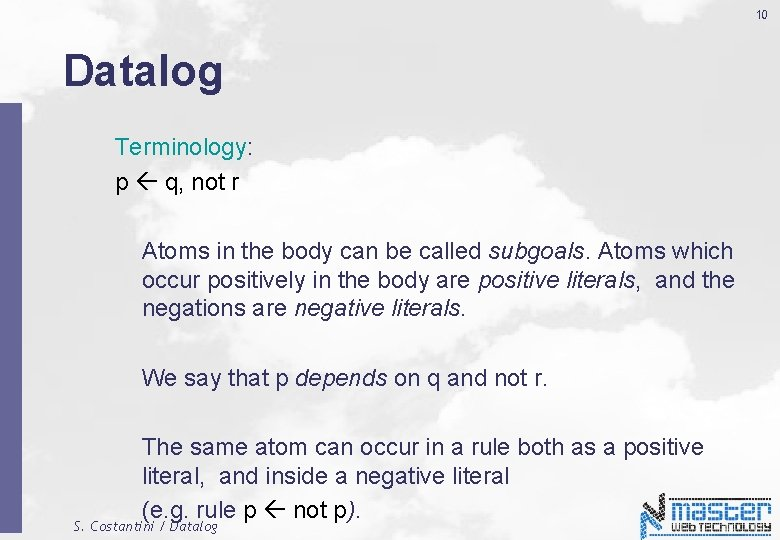10 Datalog Terminology: p q, not r Atoms in the body can be called subgoals. Atoms which occur positively in the body are positive literals, and the negations are negative literals. We say that p depends on q and not r. The same atom can occur in a rule both as a positive literal, and inside a negative literal (e. g. rule p not p). S. Costantini / Datalog11 Datalog Programs » » A Datalog theory, or “program”, is a collection of rules. In a program, predicates can be either 1. EDB = Extensional Database = facts. 2. IDB = Intensional Database = relation defined by rules. S. Costantini / Datalog12 Datalog Theory, or Program: Example p(X) r(X), q(X). r(Z) s(Z). s(a). s(b). q(b). » In every rule, each variable stands for the same value. » Thus, variables can be considered as “placeholders” for values. » Possible values are those that occur as constants in some rule/fact of the program itself. S. Costantini / Datalog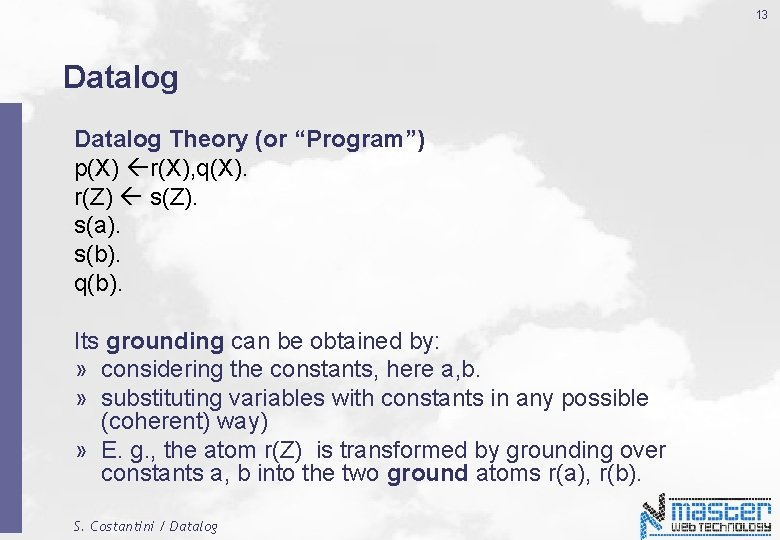13 Datalog Theory (or “Program”) p(X) r(X), q(X). r(Z) s(Z). s(a). s(b). q(b). Its grounding can be obtained by: » considering the constants, here a, b. » substituting variables with constants in any possible (coherent) way) » E. g. , the atom r(Z) is transformed by grounding over constants a, b into the two ground atoms r(a), r(b). S. Costantini / Datalog14 Grounding p(X) r(X), q(X). r(Z) s(Z). s(a). s(b). q(b). » E. g. , the atom r(Z) is transformed by grounding over constants a, b into the two ground atoms r(a), r(b). » Rule r(Z) s(Z). is transformed into the two rules r(a) s(a). r(b) s(b). S. Costantini / Datalog15 Datalog Grounding of the program p(a) r(a), q(a). p(b) r(b), q(b). r(a) s(a). r(b) s(b). s(a). s(b). q(b). Semantics: Least Herbrand Model M = {s(a), s(b), q(b), r(a), r(b), p(b)} S. Costantini / Datalog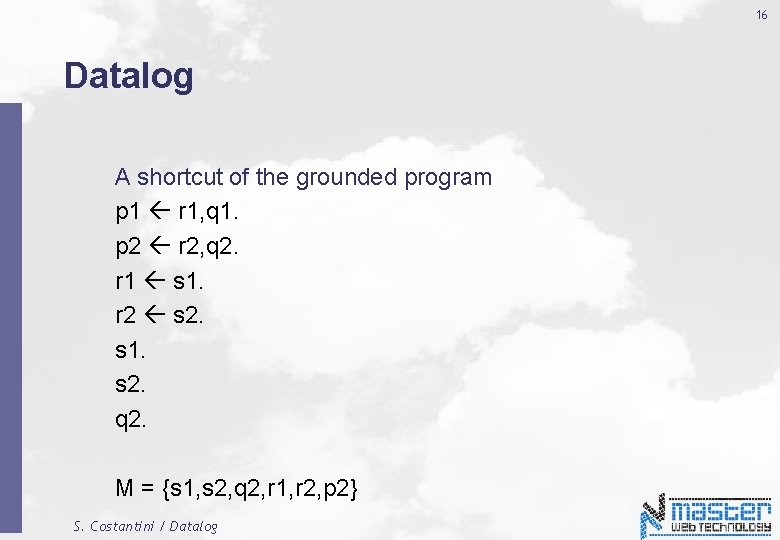16 Datalog A shortcut of the grounded program p 1 r 1, q 1. p 2 r 2, q 2. r 1 s 1. r 2 s 2. s 1. s 2. q 2. M = {s 1, s 2, q 2, r 1, r 2, p 2} S. Costantini / Datalog17 Datalog semantics » Without negation (no negative literal in the body of rules): Least Herbrand Model › The head of a rule is in the Least Herbrand Model only if the body is in the Least Herbrand Model. › The head of a rule is concluded true (or simply concluded) if all literals in its body can be concluded. › Equivalently, the head of a rule is derived if all literals in its body can be derived. » With negation: several proposals. S. Costantini / Datalog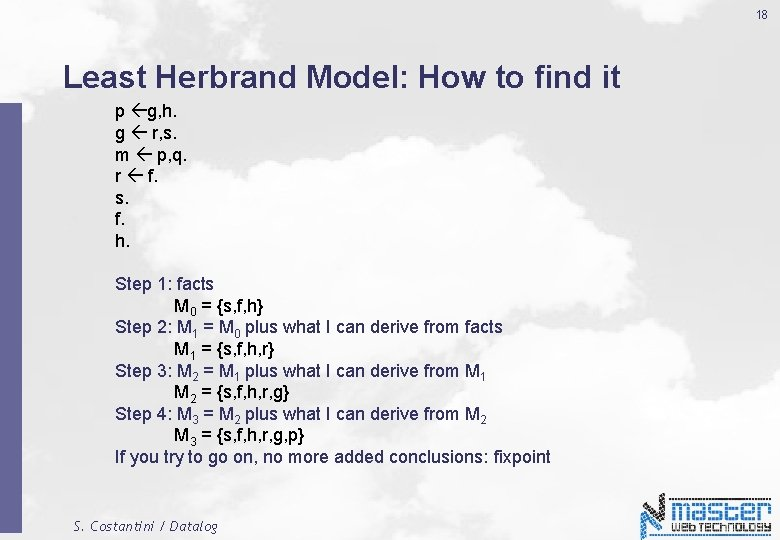18 Least Herbrand Model: How to find it p g, h. g r, s. m p, q. r f. s. f. h. Step 1: facts M 0 = {s, f, h} Step 2: M 1 = M 0 plus what I can derive from facts M 1 = {s, f, h, r} Step 3: M 2 = M 1 plus what I can derive from M 1 M 2 = {s, f, h, r, g} Step 4: M 3 = M 2 plus what I can derive from M 2 M 3 = {s, f, h, r, g, p} If you try to go on, no more added conclusions: fixpoint S. Costantini / Datalog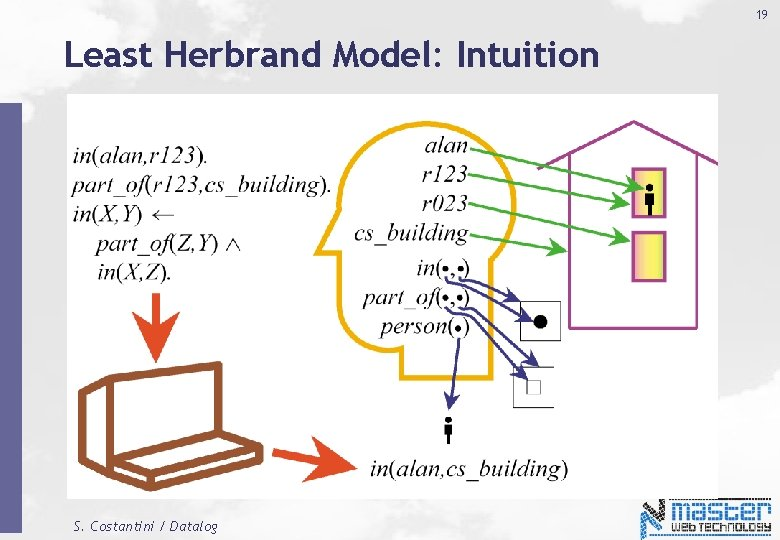19 Least Herbrand Model: Intuition S. Costantini / Datalog20 Least Herbrand Model: Intuition » Every constant and predicate of a program has an “interpretation”. » The computer cannot guess the “mental” view of a program. » Principle: do not add more than required, do not “guess” details. › Then, every predicate and constant is by default interpreted into itself. › The “minimal” intepretation is chosen. S. Costantini / Datalog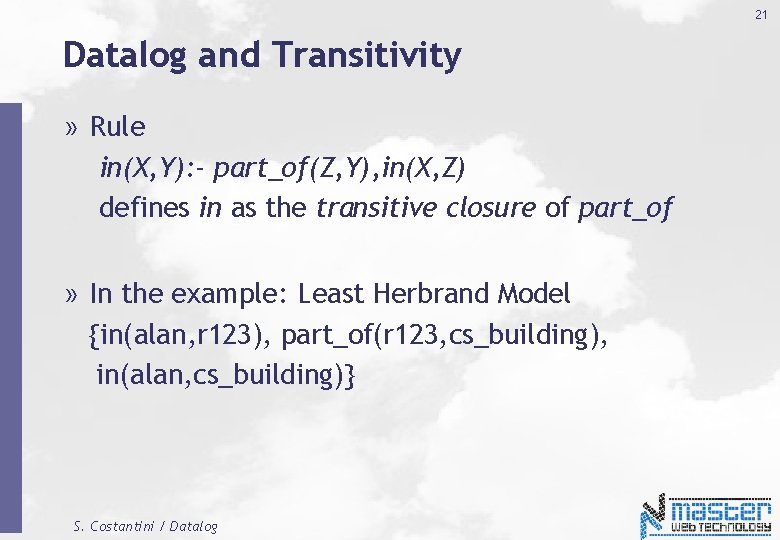21 Datalog and Transitivity » Rule in(X, Y): - part_of(Z, Y), in(X, Z) defines in as the transitive closure of part_of » In the example: Least Herbrand Model {in(alan, r 123), part_of(r 123, cs_building), in(alan, cs_building)} S. Costantini / Datalog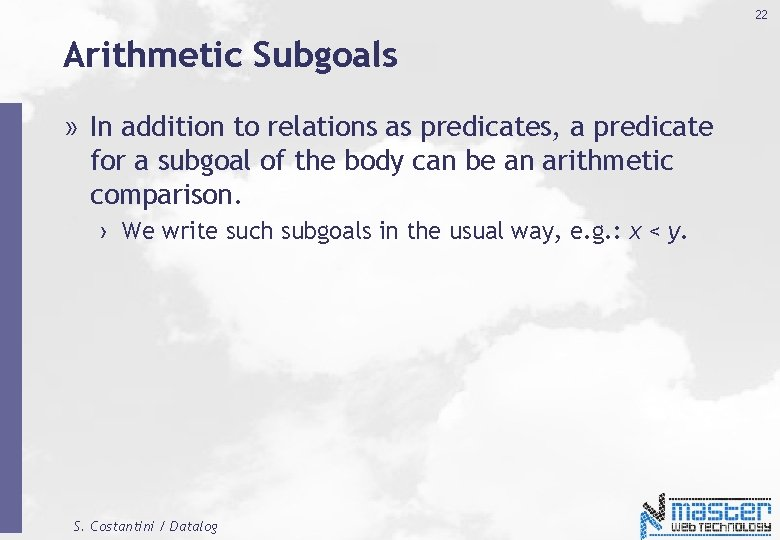22 Arithmetic Subgoals » In addition to relations as predicates, a predicate for a subgoal of the body can be an arithmetic comparison. › We write such subgoals in the usual way, e. g. : x < y. S. Costantini / Datalog23 Example: Arithmetic » A beer is “cheap” if there at least two bars that sell it for under \$2. cheap(Beer) : - sells(Bar 1, Beer, P 1), sells(Bar 2, Beer, P 2), p 1 < 2. 00 p 2 < 2. 00, bar 1 <> bar 2 S. Costantini / Datalog24 Negated Subgoals » We may put not in front of a subgoal, to negate its meaning. » Example: Think of arc(a, b) as arcs in a graph. › s(X, Y) says that: - there is a path of length 2 from X to Y - but there is no arc from X to Y. s(X, Y) : - arc(X, Z), arc(Z, Y), not arc(X, Y) S. Costantini / Datalog25 Negation » Negation in Datalog is default negation: › Let a be a ground atom, i. e. , an atom where every variable has a value. › Assume that we are not able to conclude a. › Then, we assume that not a holds. » We conclude not a by using the Closed World Assumption: all the knowledge we have is represented in the program. S. Costantini / Datalog26 Safe Rules » A rule is safe if: 1. Each distinguished variable, 2. Each variable in an arithmetic subgoal, 3. Each variable in a negated subgoal, » also appears in a nonnegated, relational subgoal. We allow only safe rules. S. Costantini / Datalog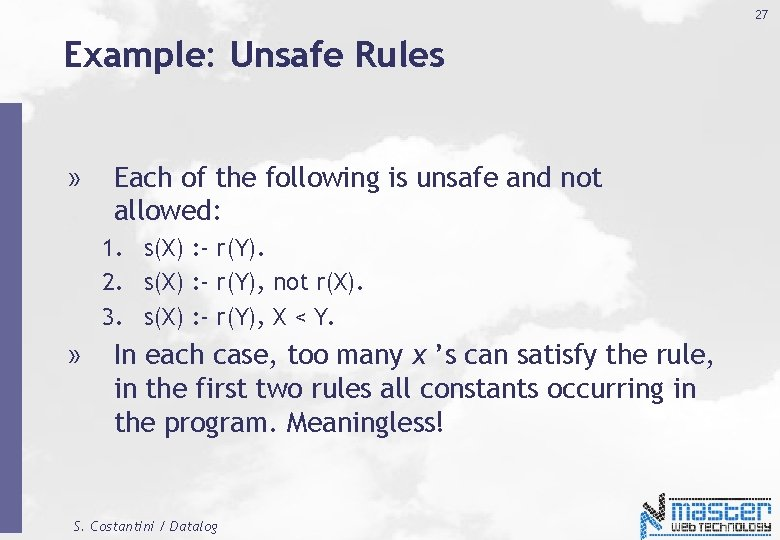27 Example: Unsafe Rules » Each of the following is unsafe and not allowed: 1. s(X) : - r(Y). 2. s(X) : - r(Y), not r(X). 3. s(X) : - r(Y), X < Y. » In each case, too many x ’s can satisfy the rule, in the first two rules all constants occurring in the program. Meaningless! S. Costantini / Datalog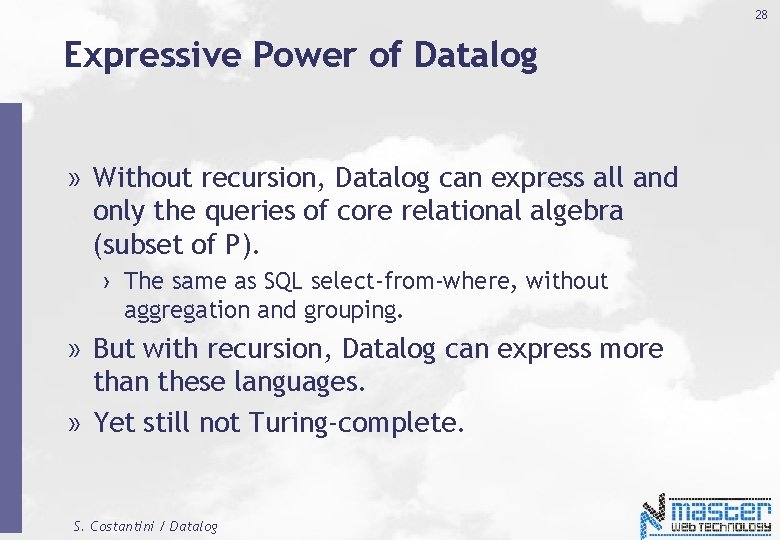28 Expressive Power of Datalog » Without recursion, Datalog can express all and only the queries of core relational algebra (subset of P). › The same as SQL select-from-where, without aggregation and grouping. » But with recursion, Datalog can express more than these languages. » Yet still not Turing-complete. S. Costantini / Datalog29 Recursion: Example parent(X, Y): - mother(X, Y). parent(X, Y): - father(X, Y). ancestor(X, Y): - parent(X, Z), ancestor(Z, Y). mother(a, b). father(c, b). mother(b, d). … other facts … » A parent X of Y is either a mother or a father (disjunction as alternative rules); » An ancestor is either a parent, or the parent of an ancestor (the ancestor X of Y is the parent of some Z who in turn is an ancestor of Y). S. Costantini / Datalog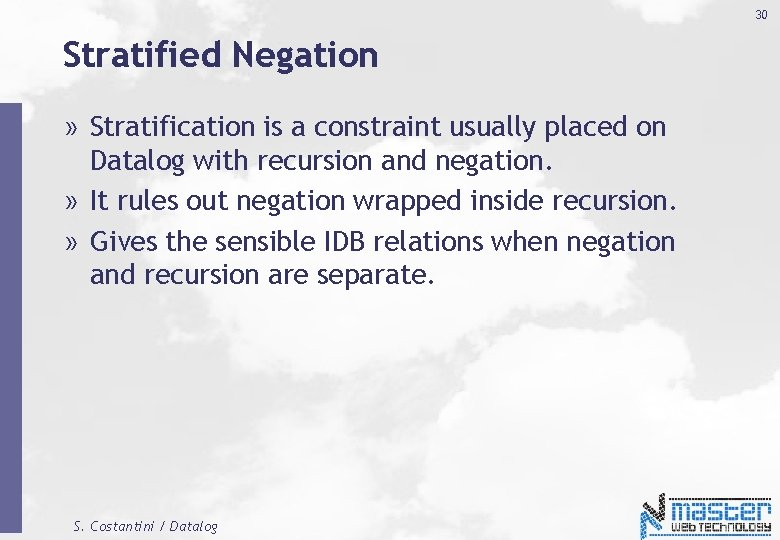30 Stratified Negation » Stratification is a constraint usually placed on Datalog with recursion and negation. » It rules out negation wrapped inside recursion. » Gives the sensible IDB relations when negation and recursion are separate. S. Costantini / Datalog31 Problematic Recursive Negation p(X) : - q(X), not p(X) q(1). q(2). Try to compute Least Herbrand Model: Initial: M = {q(1), q(2) } Round 1: M = {q(1), q(2), p(1), p(2)} Round 2: M = {q(1), q(2) } Round 3: M = {q(1), q(2), p(1), p(2)}, etc. … S. Costantini / Datalog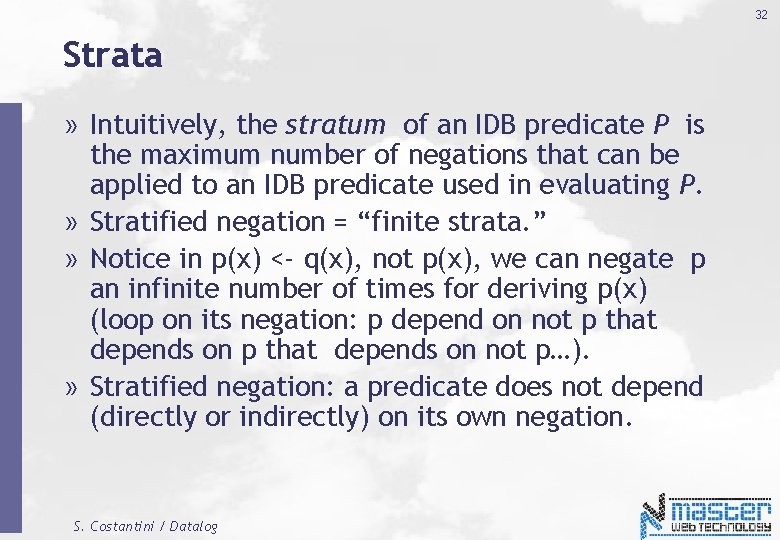32 Strata » Intuitively, the stratum of an IDB predicate P is the maximum number of negations that can be applied to an IDB predicate used in evaluating P. » Stratified negation = “finite strata. ” » Notice in p(x) <- q(x), not p(x), we can negate p an infinite number of times for deriving p(x) (loop on its negation: p depend on not p that depends on not p…). » Stratified negation: a predicate does not depend (directly or indirectly) on its own negation. S. Costantini / Datalog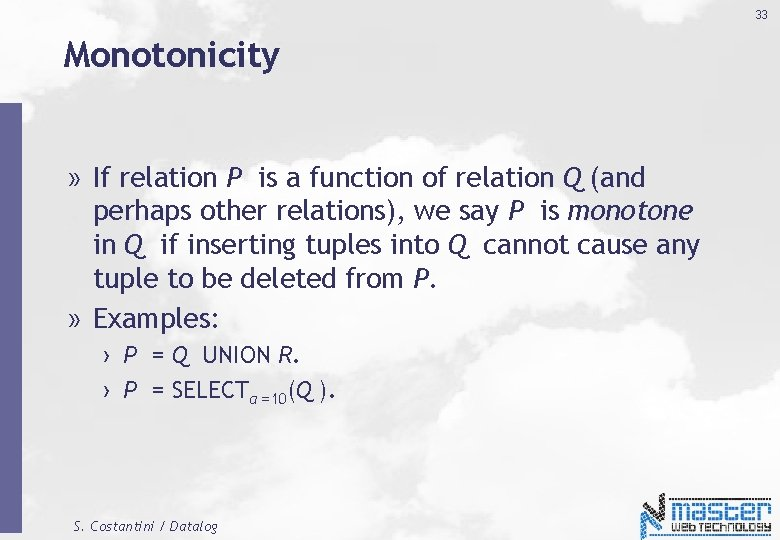33 Monotonicity » If relation P is a function of relation Q (and perhaps other relations), we say P is monotone in Q if inserting tuples into Q cannot cause any tuple to be deleted from P. » Examples: › P = Q UNION R. › P = SELECTa =10(Q ). S. Costantini / Datalog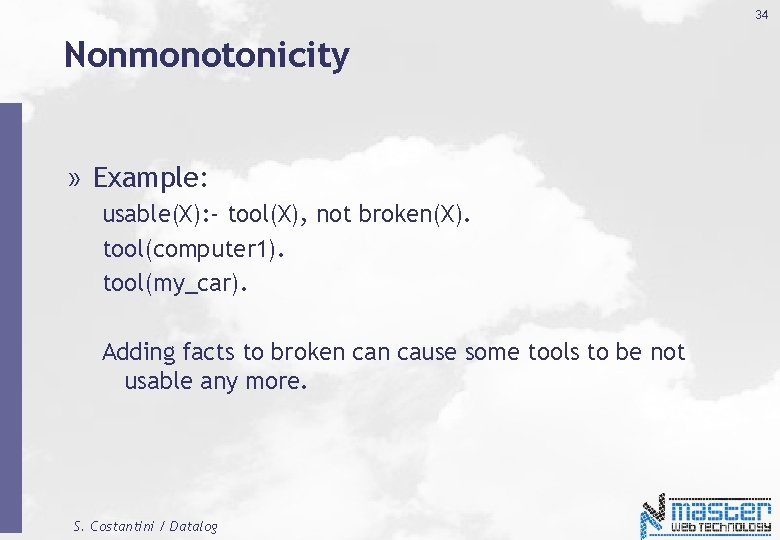34 Nonmonotonicity » Example: usable(X): - tool(X), not broken(X). tool(computer 1). tool(my_car). Adding facts to broken cause some tools to be not usable any more. S. Costantini / Datalog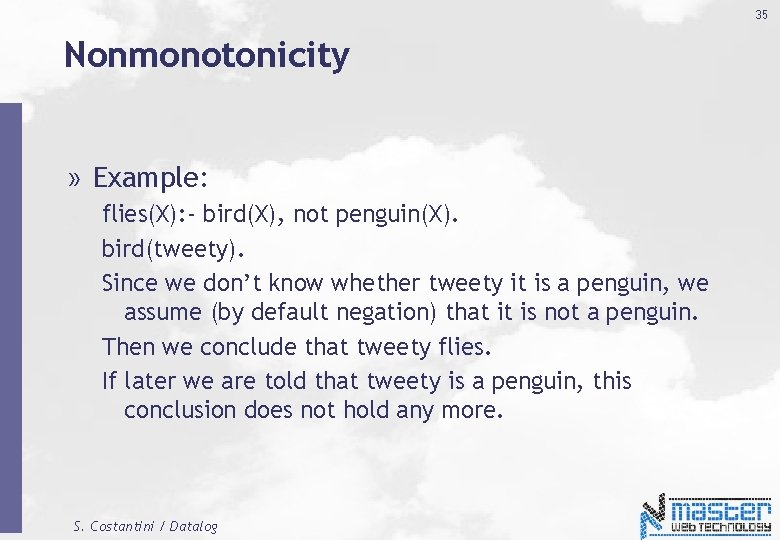35 Nonmonotonicity » Example: flies(X): - bird(X), not penguin(X). bird(tweety). Since we don’t know whether tweety it is a penguin, we assume (by default negation) that it is not a penguin. Then we conclude that tweety flies. If later we are told that tweety is a penguin, this conclusion does not hold any more. S. Costantini / Datalog36 Datalog with negation (Datalog ) » How to deal with › Unstratified negation › Nonmonotonicity » One possibility: don’t accept them » Another possibility: extend the approach (e. g. , Answer Set Semantics) S. Costantini / Datalog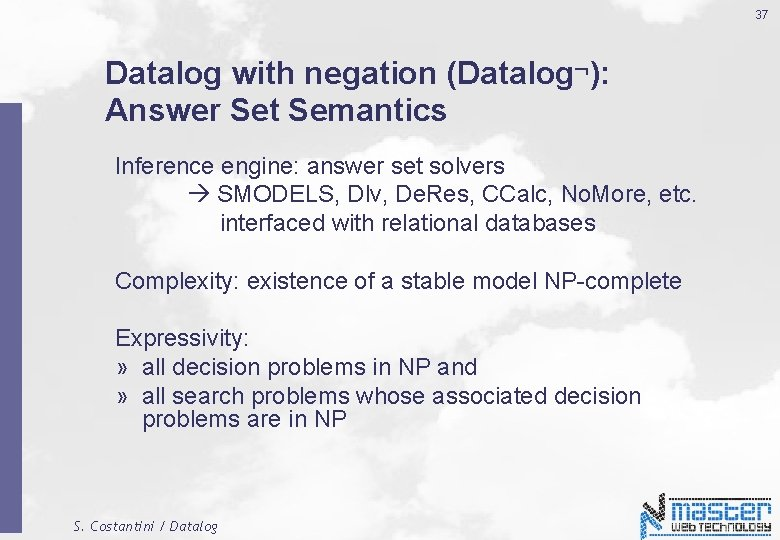37 Datalog with negation (Datalog ): Answer Set Semantics Inference engine: answer set solvers SMODELS, Dlv, De. Res, CCalc, No. More, etc. interfaced with relational databases Complexity: existence of a stable model NP-complete Expressivity: » all decision problems in NP and » all search problems whose associated decision problems are in NP S. Costantini / Datalog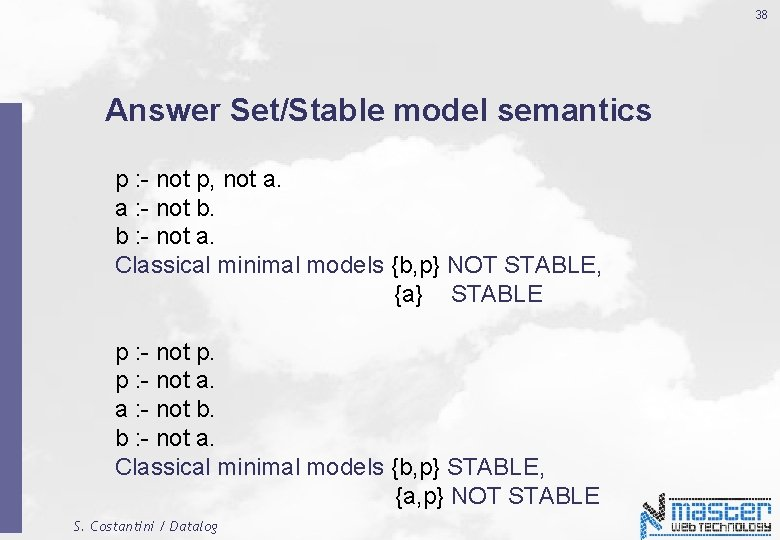38 Answer Set/Stable model semantics p : - not p, not a. a : - not b. b : - not a. Classical minimal models {b, p} NOT STABLE, {a} STABLE p : - not p. p : - not a. a : - not b. b : - not a. Classical minimal models {b, p} STABLE, {a, p} NOT STABLE S. Costantini / Datalog39 Answer Set semantics p q, not s. q. r a. r b. a not b. b not a. Classical minimal models {p, q, r, a} and {p, q, r, b}, {s, q, r, a} and {s, q, r, b}. Answer Sets (Stable Models) {p, q, r, a} and {p, q, r, b}. S. Costantini / Datalog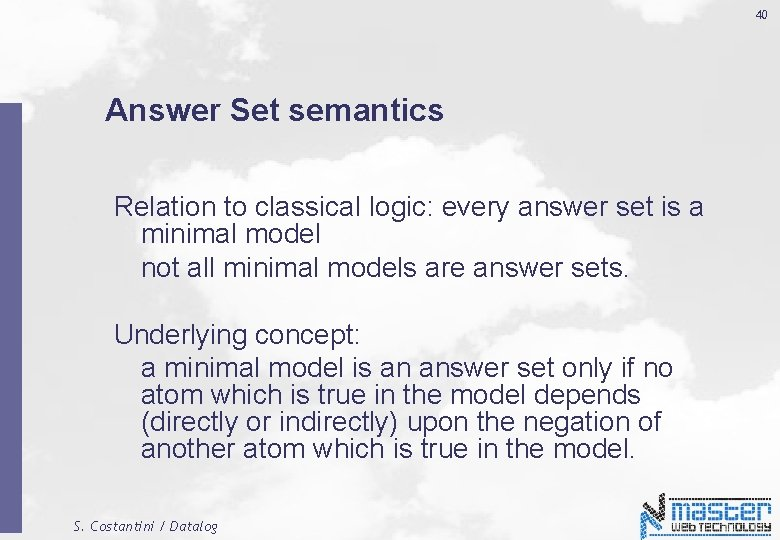40 Answer Set semantics Relation to classical logic: every answer set is a minimal model not all minimal models are answer sets. Underlying concept: a minimal model is an answer set only if no atom which is true in the model depends (directly or indirectly) upon the negation of another atom which is true in the model. S. Costantini / Datalog41 Drawbacks of Answer Set Semantics For atom A, REL_RUL(A) may have answer sets where A is true/false, while the overall program does not. p : - not p, not a. q : - not q, not b. a : - not b. b : - not a. REL_RUL(a) = {a : - not b. b : - not a. } with stable models {a} and {b}. Overall program: no stable models. S. Costantini / Datalog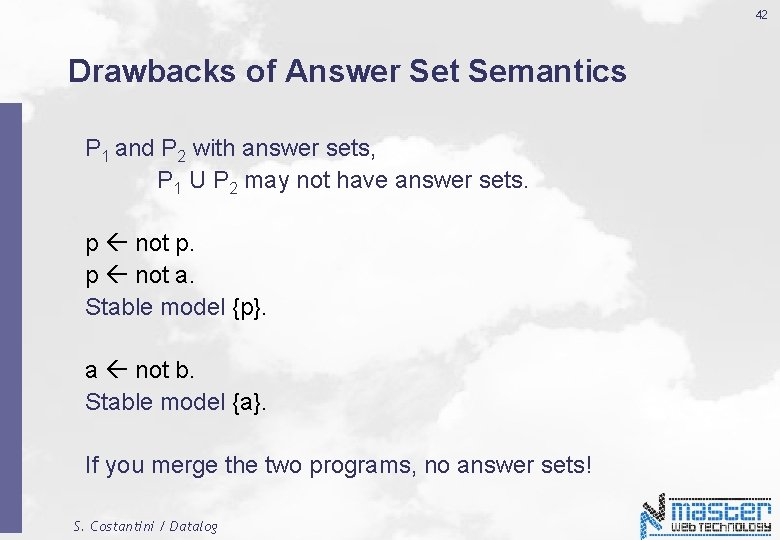42 Drawbacks of Answer Set Semantics P 1 and P 2 with answer sets, P 1 U P 2 may not have answer sets. p not p. p not a. Stable model {p}. a not b. Stable model {a}. If you merge the two programs, no answer sets! S. Costantini / Datalog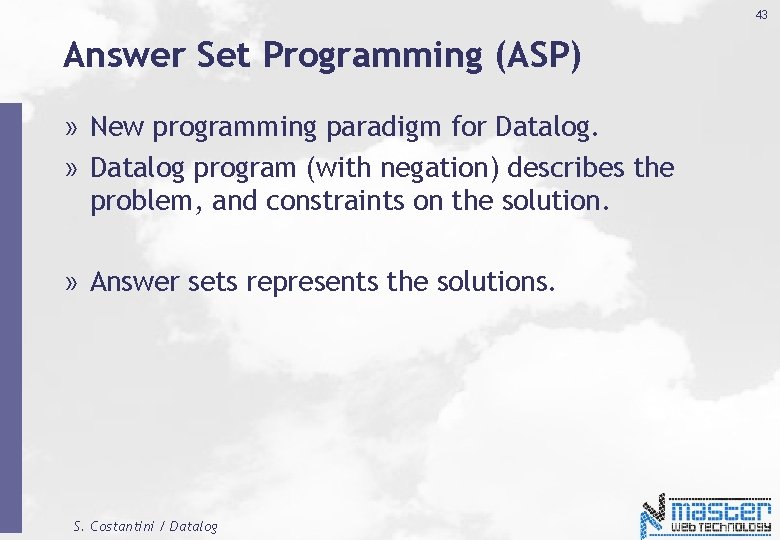43 Answer Set Programming (ASP) » New programming paradigm for Datalog. » Datalog program (with negation) describes the problem, and constraints on the solution. » Answer sets represents the solutions. S. Costantini / Datalog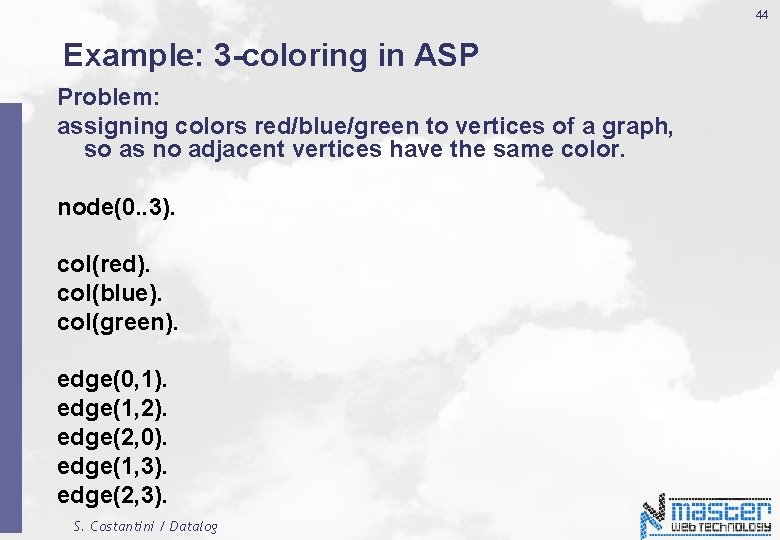44 Example: 3 -coloring in ASP Problem: assigning colors red/blue/green to vertices of a graph, so as no adjacent vertices have the same color. node(0. . 3). col(red). col(blue). col(green). edge(0, 1). edge(1, 2). edge(2, 0). edge(1, 3). edge(2, 3). S. Costantini / Datalog45 Example: 3 -coloring in Logic Programming … Inference Engine … Expected solutions: color(0, red), color(1, blue), color(2, green), color(3, red) color(0, red), color(1, green), color(2, blue), color(3, red) color(0, blue), color(1, red), color(2, green), color(3, blue) color(0, blue), color(1, green), color(2, red), color(3, blue) color(0, green), color(1, blue), color(2, red), color(3, green) color(0, green), color(1, red), color(2, blue), color(3, green) S. Costantini / Datalog46 3 -coloring in Answer Set Programming color(X, red) | color(X, blue) | color(X, green) : - node(X). : - edge(X, Y), col(C), color(X, C), color(Y, C). S. Costantini / Datalog47 3 -coloring in Answer Set Programming Using the SMODELS inference engine we obtain: lparse < 3 col. txt | smodels 0 Answer 1 color(0, red), color(1, blue), color(green), color(3, red) Answer 2 color(0, red), color(1, green), color(blue), color(3, red) … S. … Costantini / Datalog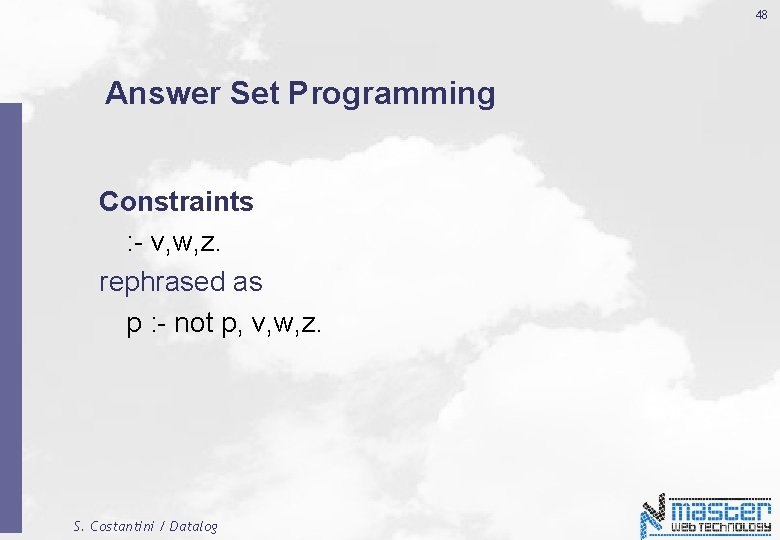48 Answer Set Programming Constraints : - v, w, z. rephrased as p : - not p, v, w, z. S. Costantini / Datalog49 Answer Set Programming Disjunction v | w |z. rephrased as v : - not w, not z. w : - not v, not z. z : - not v, not w. S. Costantini / Datalog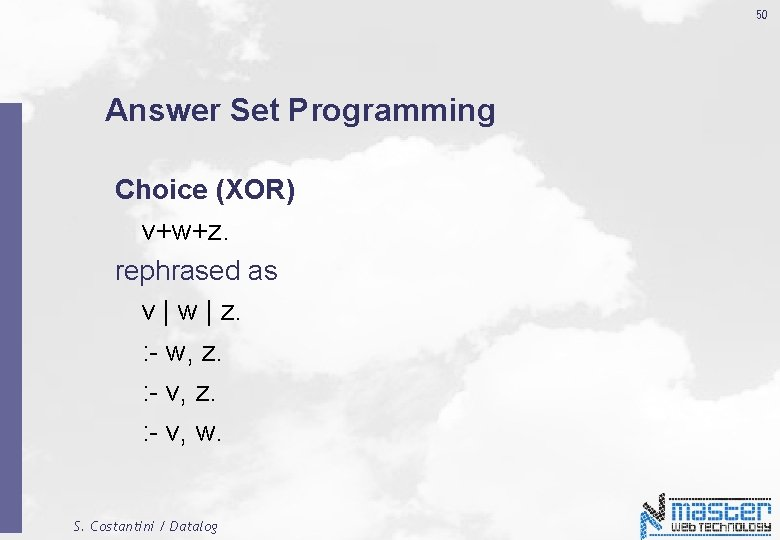50 Answer Set Programming Choice (XOR) v+w+z. rephrased as v | w | z. : - w, z. : - v, w. S. Costantini / Datalog51 Answer Set Programming Classical Negation ¬p - p : - q, r. rephrased as p' : - q, r. : - p, p‘ S. Costantini / Datalog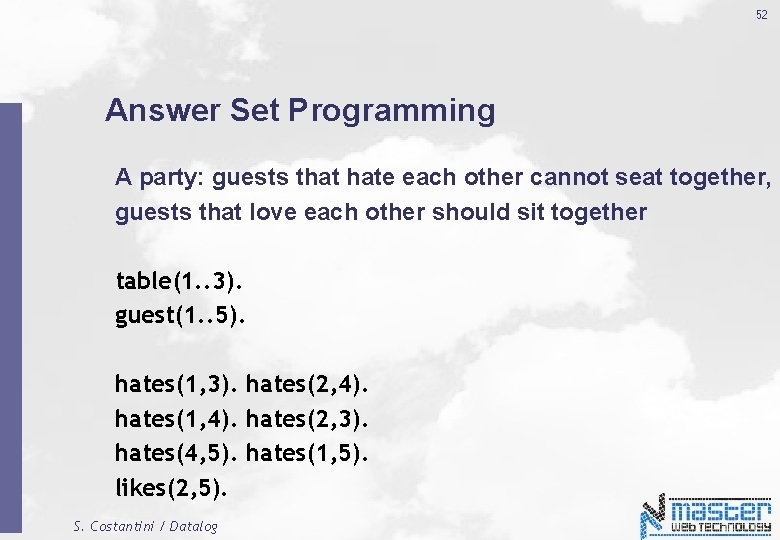52 Answer Set Programming A party: guests that hate each other cannot seat together, guests that love each other should sit together table(1. . 3). guest(1. . 5). hates(1, 3). hates(2, 4). hates(1, 4). hates(2, 3). hates(4, 5). hates(1, 5). likes(2, 5). S. Costantini / Datalog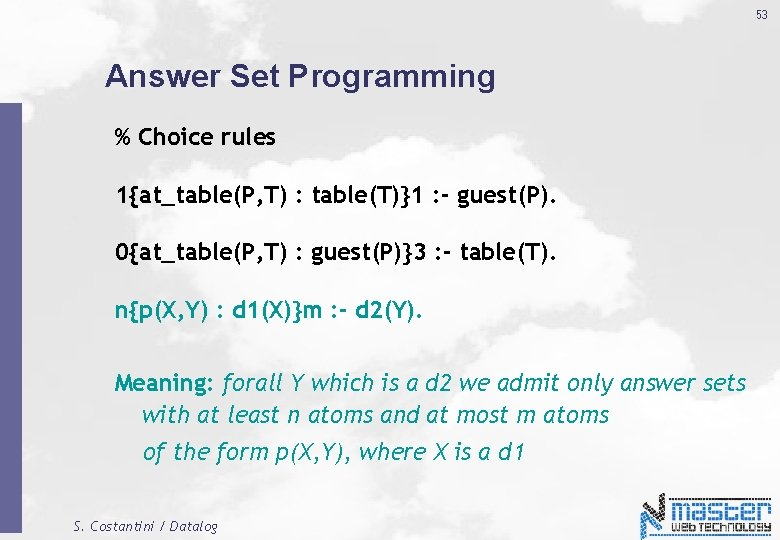53 Answer Set Programming % Choice rules 1{at_table(P, T) : table(T)}1 : - guest(P). 0{at_table(P, T) : guest(P)}3 : - table(T). n{p(X, Y) : d 1(X)}m : - d 2(Y). Meaning: forall Y which is a d 2 we admit only answer sets with at least n atoms and at most m atoms of the form p(X, Y), where X is a d 1 S. Costantini / Datalog54 Answer Set Programming % hard constraint : - hates(P 1, P 2), at_table(P 1, T), at_table(P 2, T), guest(P 1), guest(P 2), table(T). % should be a soft constraint! : - likes(P 1, P 2), at_table(P 1, T 1), at_table(P 2, T 2), T 1 != T 2, guest(P 1), guest(P 2), table(T 1), table(T 2). S. Costantini / Datalog55 S. Costantini / Datalog